x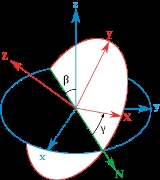Euler anglesOverview

The Euler angles are three angles introduced by Leonhard Euler
Leonhard Euler
Leonhard Euler was a pioneering Swiss mathematician and physicist. He made important discoveries in fields as diverse as infinitesimal calculus and graph theory. He also introduced much of the modern mathematical terminology and notation, particularly for mathematical analysis, such as the notion...

to describe the orientation
Orientation (geometry)
In geometry the orientation, angular position, or attitude of an object such as a line, plane or rigid body is part of the description of how it is placed in the space it is in....

of a rigid body
Rigid body
In physics, a rigid body is an idealization of a solid body of finite size in which deformation is neglected. In other words, the distance between any two given points of a rigid body remains constant in time regardless of external forces exerted on it...

. To describe such an orientation in 3-dimensional
Dimension
In physics and mathematics, the dimension of a space or object is informally defined as the minimum number of coordinates needed to specify any point within it. Thus a line has a dimension of one because only one coordinate is needed to specify a point on it...

Euclidean space
Euclidean space
In mathematics, Euclidean space is the Euclidean plane and three-dimensional space of Euclidean geometry, as well as the generalizations of these notions to higher dimensions...

three parameters are required. They can be given in several ways, Euler angles being one of them; see charts on SO(3)
Charts on SO(3)
In mathematics, the special orthogonal group in three dimensions, otherwise known as the rotation group SO, is a naturally occurring example of a manifold. The various charts on SO set up rival coordinate systems: in this case there cannot be said to be a preferred set of parameters describing a...

for others.

Euler angles also represent three composed rotations that move a reference frame to a given referred frame. This is equivalent to saying that any orientation can be achieved by composing three elemental rotation
Rotation
A rotation is a circular movement of an object around a center of rotation. A three-dimensional object rotates always around an imaginary line called a rotation axis. If the axis is within the body, and passes through its center of mass the body is said to rotate upon itself, or spin. A rotation...

s (rotations around a single axis), and also equivalent to saying that any rotation matrix can be decomposed
Matrix decomposition
In the mathematical discipline of linear algebra, a matrix decomposition is a factorization of a matrix into some canonical form. There are many different matrix decompositions; each finds use among a particular class of problems.- Example :...

as a product of three elemental rotation matrices.

Without considering the possibilities of different signs for the angles or moving the reference frame, there are twelve different conventions divided in two groups.Unanswered QuestionsEncyclopedia
The Euler angles are three angles introduced by Leonhard Euler
Leonhard Euler
Leonhard Euler was a pioneering Swiss mathematician and physicist. He made important discoveries in fields as diverse as infinitesimal calculus and graph theory. He also introduced much of the modern mathematical terminology and notation, particularly for mathematical analysis, such as the notion...

to describe the orientation
Orientation (geometry)
In geometry the orientation, angular position, or attitude of an object such as a line, plane or rigid body is part of the description of how it is placed in the space it is in....

of a rigid body
Rigid body
In physics, a rigid body is an idealization of a solid body of finite size in which deformation is neglected. In other words, the distance between any two given points of a rigid body remains constant in time regardless of external forces exerted on it...

. To describe such an orientation in 3-dimensional
Dimension
In physics and mathematics, the dimension of a space or object is informally defined as the minimum number of coordinates needed to specify any point within it. Thus a line has a dimension of one because only one coordinate is needed to specify a point on it...

Euclidean space
Euclidean space
In mathematics, Euclidean space is the Euclidean plane and three-dimensional space of Euclidean geometry, as well as the generalizations of these notions to higher dimensions...

three parameters are required. They can be given in several ways, Euler angles being one of them; see charts on SO(3)
Charts on SO(3)
In mathematics, the special orthogonal group in three dimensions, otherwise known as the rotation group SO, is a naturally occurring example of a manifold. The various charts on SO set up rival coordinate systems: in this case there cannot be said to be a preferred set of parameters describing a...

for others.

Euler angles also represent three composed rotations that move a reference frame to a given referred frame. This is equivalent to saying that any orientation can be achieved by composing three elemental rotation
Rotation
A rotation is a circular movement of an object around a center of rotation. A three-dimensional object rotates always around an imaginary line called a rotation axis. If the axis is within the body, and passes through its center of mass the body is said to rotate upon itself, or spin. A rotation...

s (rotations around a single axis), and also equivalent to saying that any rotation matrix can be decomposed
Matrix decomposition
In the mathematical discipline of linear algebra, a matrix decomposition is a factorization of a matrix into some canonical form. There are many different matrix decompositions; each finds use among a particular class of problems.- Example :...

as a product of three elemental rotation matrices.

Without considering the possibilities of different signs for the angles or moving the reference frame, there are twelve different conventions divided in two groups. One of them is called "proper" Euler angles and the other Tait–Bryan angles. Sometimes "Euler angles" is used for all of them.

## Definition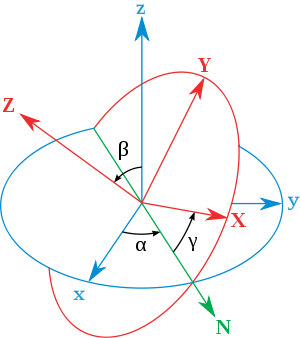Euler angles are a means of representing the spatial orientation of any frame
Basis (linear algebra)
In linear algebra, a basis is a set of linearly independent vectors that, in a linear combination, can represent every vector in a given vector space or free module, or, more simply put, which define a "coordinate system"...

(coordinate system) as a composition of rotations from a frame of reference (coordinate system). In the following, the fixed system is denoted in lower case (x,y,z) and the rotated system is denoted in upper case letters (X,Y,Z).

The definition is Static. Given a reference frame and the one whose orientation we want to describe, first we define the line of nodes (N) as the intersection of the xy and the XY coordinate planes (in other words, line of nodes is the line perpendicular to both z and Z axis). Then we define its Euler angles as:
• α (or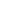) is the angle between the x-axis and the line of nodes.
• β (or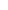) is the angle between the z-axis and the Z-axis.
• γ (or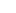) is the angle between the line of nodes and the X-axis.

Euler angles between two frames are defined only if both frames have the same handedness
Orientation (mathematics)
In mathematics, orientation is a notion that in two dimensions allows one to say when a cycle goes around clockwise or counterclockwise, and in three dimensions when a figure is left-handed or right-handed. In linear algebra, the notion of orientation makes sense in arbitrary dimensions...

. Euler angles are just one of the several ways
Charts on SO(3)
In mathematics, the special orthogonal group in three dimensions, otherwise known as the rotation group SO, is a naturally occurring example of a manifold. The various charts on SO set up rival coordinate systems: in this case there cannot be said to be a preferred set of parameters describing a...

of specifying the relative orientation of two such coordinate systems. Different authors may use different sets of angles to describe these orientations, or different names for the same angles, leading to different conventions. Therefore any discussion employing Euler angles should always be preceded by their definition.

Unless otherwise stated, this article will use the convention described in the adjacent drawing, usually named Z-X’-Z’’.

### Angles signs and ranges

Normally, angles are defined in such a way that they are positive when they rotate counter-clockwise (how they rotate depends on which side of the rotation plane we observe them from. The positive side will be the one of the positive axis of rotation)

About the ranges:
• α and γ range are defined modulo
Modular arithmetic
In mathematics, modular arithmetic is a system of arithmetic for integers, where numbers "wrap around" after they reach a certain value—the modulus....

2π radian
Radian
Radian is the ratio between the length of an arc and its radius. The radian is the standard unit of angular measure, used in many areas of mathematics. The unit was formerly a SI supplementary unit, but this category was abolished in 1995 and the radian is now considered a SI derived unit...

s. A valid range could be (−π, π].
• β range covers π radians (but can't be said to be modulo π). For example could be [0, π] or [−π/2, π/2].

The angles α, β and γ are uniquely determined except for the singular case that the xy and the XY planes are identical, the z axis and the Z axis having the same or opposite directions. Indeed, if the z-axis and the Z-axis are the same, β = 0 and only (α + γ) is uniquely defined (not the individual values), and, similarly, if the z-axis and the Z-axis are opposite, β = π and only (α − γ) is uniquely defined (not the individual values). These ambiguities are known as gimbal lock
Gimbal lock
Gimbal lock is the loss of one degree of freedom in a three-dimensional space that occurs when the axes of two of the three gimbals are driven into a parallel configuration, "locking" the system into rotation in a degenerate two-dimensional space....

in applications.

### Conventions

There are two main types of conventions called "proper" Euler angles and Tait–Bryan angles, after Peter Guthrie Tait
Peter Guthrie Tait
Peter Guthrie Tait FRSE was a Scottish mathematical physicist, best known for the seminal energy physics textbook Treatise on Natural Philosophy, which he co-wrote with Kelvin, and his early investigations into knot theory, which contributed to the eventual formation of topology as a mathematical...

and George H. Bryan
George H. Bryan
George Hartley Bryan FRS, generally referred to in the technical literature as G. H. Bryan, was a professor at University College, Bangor, Wales who is generally credited with developing the modern mathematical treatment of the motion of airplanes in flight as rigid bodies with six degrees of freedom...

, also known as Nautical or Cardan angles, after Cardan.

Their static difference is the definition for the line of nodes. In the first case two homologous planes (planes overlapping when the angles are zero) are used. In the second one, they are replaced by non-homologous planes (perpendicular when angles are zero).

Nevertheless, it is unusual to use static conventions when speaking about Euler angles. The intrinsic rotations equivalence or the extrinsic rotations equivalence are used instead. According with these equivalences, proper Euler angles are equivalent to three combined rotations repeating exactly one axis. Tait–Bryan angles are equivalent to three composed rotations in different axes.

No information is lost when using the rotation equivalence because the static parameters can be calculated from the name of the convention. For example, given the convention X-Y’-Z’’, the first rotation is perpendicular to "x" and the third one to "Z". Therefore the planes are the yz and the XY, and the line of nodes is the intersection of these two.

#### Proper Euler angles

There are six possibilities of choosing the proper Euler angles. Using the static definition they correspond to the three possible homogeneous combinations of planes (XY, XZ and YZ) with the two possible options to measure the angles from (given the line of nodes by the XY planes for example, it can be taken X-N or Y-N as first angle). Hence the six possibilities.

There is an intrinsic rotations equivalence which is normally used to name the possible conventions of Euler Angles. If we are told that some angles are given using the convention Z-X’-Z’’, this means that they are equivalent to three concatenated intrinsic rotations around some moving axes Z, X’ and Z’’ in that order. This composition is non-commutative. It has to be applied in such a way that in the beginning one of the intrinsic axis moves together with the line of nodes. The above diagram convention is usually named this way.

Nevertheless, sometimes the extrinsic rotations equivalence could be used. If this is the case, the given angles are backwards, meaning that the first angle is the intrinsic rotation and the last one the precession. The name of the convention would be indistinguishable from the previous one, even if the angles' order is the opposite, being something like z-x-z (here lowercase is used to remark extrinsic composition).

To specify that the given order means intrinsic composition, sometimes a similar notation is used, but stating explicitly which rotation axis are different for each step, as in Z-X’-Z’’. Using this notation, Z-X-Z would mean extrinsic composition.

#### Tait–Bryan angles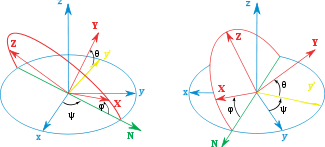There are also six Tait–Bryan combinations. They come from the two possible non-homogeneous planes that exist when one is given (given XY, there are two non-homogeneous, XZ and YZ). The three possible planes at the reference frame multiplied by the two options for each one yield the six possible conventions.

There are six possible combinations of this kind, and all of them behave in an identical way. Using the intrinsic rotations equivalence, Tait–Bryan angles correspond with the three rotations with a different axis. Z–X–Y for example. There are also six possibilities of this kind. The enclosed image shows the ZXY convention. The other five proper conventions are obtained by selecting different axes of rotation.

These three angles are normally called "Heading, Elevation and Bank", or "Yaw, Pitch and Roll". The second terms have to be used carefully because they are also the names for the three aircraft principal axes
Aircraft principal axes
An aircraft in flight is free to rotate in three dimensions: pitch, nose up or down about an axis running from wing to wing), yaw, nose left or right about an axis running up and down; and roll, rotation about an axis running from nose to tail. The axes are alternatively designated as lateral,...

.

For Tait–Bryan angles, also intrinsic and extrinsic conventions can be used, giving therefore two meanings for every convention name. For example, X–Y–Z, using intrinsic convention, means that a X-rotation is performed, composing intrinsic rotations Y and Z later, but using extrinsic convention means that after the X rotation, extrinsic rotations Y and Z are performed. The meaning is different in both cases.

#### Conversion between extrinsic and intrinsic conventions

For either Euler or Tait-Bryan angles, it is very simple to convert from an intrinsic (rotating axes) to an extrinsic (static axes) convention, and vice-versa: just swap the order of the operations. An (α, β, γ) rotation using X-Y-Z intrinsic convention is equivalent to a (γ, β, α) rotation using Z-Y-X extrinsic convention; this is true for all Euler or Tait-Bryan axis combinations.

## Geometric derivation

The fastest way to get the Euler Angles of a given frame is to write the three given vectors as columns of a matrix and compare it with the expression of the theoretical matrix (see later table of matrices). Hence the three Euler Angles can be calculated.

Nevertheless, the same result can be reached avoiding matrix algebra, which is more geometrical. Assuming a frame with unitary vectors (X, Y, Z) as in the main diagram, it can be seen that: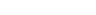and since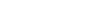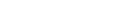as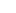is a double projection of an unitary vector: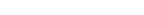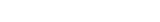There is a similar construction for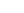, projecting it first over the plane defined by the axis Z and the line of nodes. As the angle between the planes is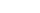and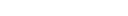, this leads to: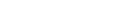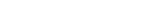and finally, using the cosine inverse function arc cos: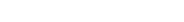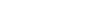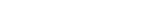It is interesting to note that the cosine inverse function yields two possible values for the argument. In this geometrical description only one of the solutions is valid. When Euler angles are defined as a sequence of rotations all the solutions can be valid, but there will be only one inside the angles ranges. This is because the sequence of rotations to reach the target frame is not unique if the ranges are not previously defined.

For computational purposes, it may be useful to represent the angles using atan2
Atan2
In trigonometry, the two-argument function atan2 is a variation of the arctangent function. For any real arguments and not both equal to zero, is the angle in radians between the positive -axis of a plane and the point given by the coordinates on it...

(y,x):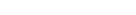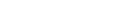## Relationship with physical motions

See also Givens rotations

Euler angles can be considered as the result of three composed rotations and these conventions are named according to this composition. Since intrinsic rotations produce the same result as extrinsic rotations inverted, there are two possible names for any static convention. For example the intrinsic ZXY and the extrinsic yxz have the same static parameters.

### Euler angles as composition of intrinsic rotations

Starting with an initial set of mobile axes, say XYZ overlapping the reference axes xyz, a composition of three intrinsic rotations (rotations only about the mobile frame axes
Moving frame
In mathematics, a moving frame is a flexible generalization of the notion of an ordered basis of a vector space often used to study the extrinsic differential geometry of smooth manifolds embedded in a homogeneous space.-Introduction:...

, assuming active composition
Active and passive transformation
In the physical sciences, an active transformation is one which actually changes the physical position of a system, and makes sense even in the absence of a coordinate system whereas a passive transformation is a change in the coordinate description of the physical system . The distinction between...

) can be used to reach any target frame with an origin coincident with that of XYZ from the reference frame. The value of the rotations are the Euler Angles.

The position of the mobile axes can be reached using three rotations with angles α, β, γ in three ways equivalent to the former definition, as follows:

The XYZ system rotates while the xyz is fixed. Starting with the XYZ system overlapping the reference frame xyz, the same rotations as before can be performed using only rotations around the mobile axes XYZ.
• Rotate the XYZ-system about the Z-axis by α. The X-axis now lies on the line of nodes.
• Rotate the XYZ-system again about the now rotated X-axis by β. The Z-axis is now in its final orientation, and the x-axis remains on the line of nodes.
• Rotate the XYZ-system a third time about the new Z-axis by γ.

Any convention for proper Euler angles is equivalent to three such rotations, with one axis being repeated (ZXZ for example). Tait-Bryan angles are also equivalent to three composed rotations, but in this case, all three rotations are around different axes (ZXY).

Usually conventions are named according with this equivalence.

### Euler angles as composition of extrinsic rotations

Also composition of extrinsic rotations (rotations about the reference frame axes, assuming active composition
Active and passive transformation
In the physical sciences, an active transformation is one which actually changes the physical position of a system, and makes sense even in the absence of a coordinate system whereas a passive transformation is a change in the coordinate description of the physical system . The distinction between...

) can be used to reach any target frame. Let xyz system be fixed while the XYZ system rotates. Start with the rotating XYZ system coinciding with the fixed xyz system.
• Rotate the XYZ-system about the z-axis by γ. The X-axis is now at angle γ with respect to the x-axis.
• Rotate the XYZ-system again about the x-axis by β. The Z-axis is now at angle β with respect to the z-axis.
• Rotate the XYZ-system a third time about the z-axis by α. The first and third axes are identical.

This can be shown to be equivalent to the previous statement:

Let us call (e), (f), (g), (h), the successive frames deduced from the initial (e) reference frame by the successive intrinsic rotations described above. We call u, v, w, t, the successive vectors obtained with that rotation. We write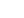for the column matrix representing a vector x in the frame (e). If necessary we add also a lower index to any matrix we wish to operate in a specific frame. We call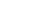,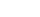,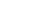the successive rotations of our example. Thus we can write when describing the intrinsic operations :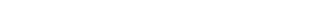When describing the intrinsic rotations in the (e) reference frame we must of course transform the matrices used to represent the rotations. Then by the rules of matrix algebra we get :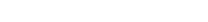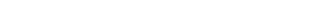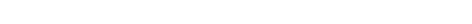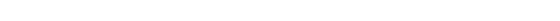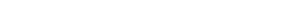The relation (5) can then of course be interpreted in extrinsic manner as a succession of rotations around the (e) axes.

Again, proper Euler angles repeat an axis and Tait–Bryan angles do not. As before, this kind of composition is non-commutative.

### Euler rotations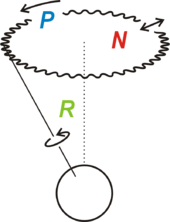Euler rotations are defined as the movement obtained by changing one of the Euler angles while leaving the other two constant. Euler rotations are never expressed in terms of the external frame, or in terms of the co-moving rotated body frame, but in a mixture. They constitute a mixed axes of rotation system, where the first angle moves the line of nodes around the external axis z, the second rotates around the line of nodes and the third one is an intrinsic rotation around an axis fixed in the body that moves.

These rotations are called Precession
Precession
Precession is a change in the orientation of the rotation axis of a rotating body. It can be defined as a change in direction of the rotation axis in which the second Euler angle is constant...

, Nutation
Nutation
Nutation is a rocking, swaying, or nodding motion in the axis of rotation of a largely axially symmetric object, such as a gyroscope, planet, or bullet in flight, or as an intended behavior of a mechanism...

, and intrinsic rotation
Rotation
A rotation is a circular movement of an object around a center of rotation. A three-dimensional object rotates always around an imaginary line called a rotation axis. If the axis is within the body, and passes through its center of mass the body is said to rotate upon itself, or spin. A rotation...

. While they are rotations when they are applied over individual frames, only precession is valid as a rotation operator, and only precession can be expressed in general as a matrix in the basis of the space.

#### Gimbal analogy

If we suppose a set of frames, able to move each with respect to the former according to just one angle, like a gimbal, there will exist an external fixed frame, one final frame and two frames in the middle, which are called "intermediate frames". The two in the middle work as two gimbal rings that allow the last frame to reach any orientation in space.

#### Intermediate frames

The gimbal rings indicate some intermediate frames. They can be defined statically too. Taking some vectors i, j and k over the axes x, y and z, and vectors I, J, K over X, Y and Z, and a vector N over the line of nodes, some intermediate frames can be defined using the vector cross product
Cross product
In mathematics, the cross product, vector product, or Gibbs vector product is a binary operation on two vectors in three-dimensional space. It results in a vector which is perpendicular to both of the vectors being multiplied and normal to the plane containing them...

, as following:
• origin: [i,j,k] (where k = i × j)
• first: [N,k × N,k]
• second: [N,K × N,K]
• final: [I,J,K]

These intermediate frames are equivalent to those of the gimbal. They are such that they differ from the previous one in just a single elemental rotation. This proves that:
• Any target frame can be reached from the reference frame just composing three rotations.
• The values of these three rotations are exactly the Euler angles of the target frame.

## Relationship to other representations

Euler angles are one way to represent orientations. There are others, and it is possible to change to and from other conventions.

### Matrix orientation

Using the equivalence between Euler angles and rotation composition, it is possible to change to and from matrix convention.

Fixed (world) axes and column vectors, with intrinsic composition (composition of rotations about body axes) of active rotations
Active and passive transformation
In the physical sciences, an active transformation is one which actually changes the physical position of a system, and makes sense even in the absence of a coordinate system whereas a passive transformation is a change in the coordinate description of the physical system . The distinction between...

and the right-handed rule for the positive sign of the angles are assumed. This means for example that a convention named (YXZ) is the result of performing first an intrinsic Z rotation, followed by X and Y rotations, in the moving axes (Note: For intrinsic rotations compositions, the order of multiplication of matrices is the opposite of the order in which they're applied to a vector). Its matrix is the product of Rot(Y1) Rot(X2) Rot(Z3) like this: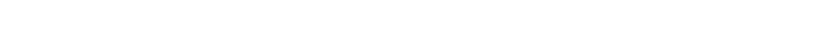.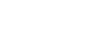.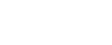Subindexes refer to the order in which the angles are applied. Trigonometric notation has been simplified. For example, c1 means cos(θ1) and s2 means sin(θ2). As we assumed intrinsic and active compositions, θ1 is the external angle of the static definition (angle between fixed axis x and line of nodes) and θ3 the internal angle (from the line of nodes to rotated axis X). The following tables can be used both ways, to obtain an orientation matrix from Euler angles and to obtain Euler angles from the matrix. The possible combinations of rotations equivalent to Euler angles are shown here. One table for the left-handed (used for example in computer graphics) convention for the positive sign and one for the right-handed (used for example in dynamics) convention.

Left-handed positive sign convention
XZX XZY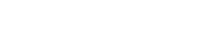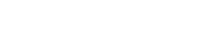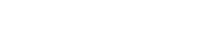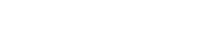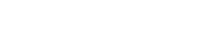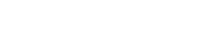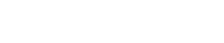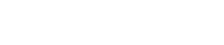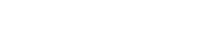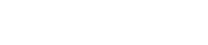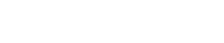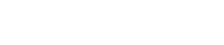Right-handed positive sign convention
XZX XZY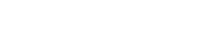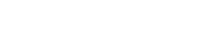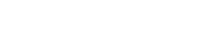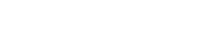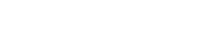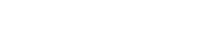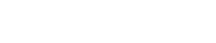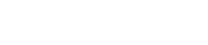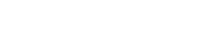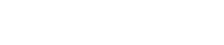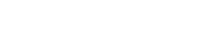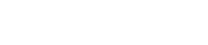### Quaternions

Unit quaternions, also known as Euler–Rodrigues parameters, provide another mechanism
Quaternions and spatial rotation
Unit quaternions provide a convenient mathematical notation for representing orientations and rotations of objects in three dimensions. Compared to Euler angles they are simpler to compose and avoid the problem of gimbal lock. Compared to rotation matrices they are more numerically stable and may...

for representing 3D rotations. This is equivalent to the special unitary group description.

Expressing rotations in 3D as unit quaternions instead of matrices has some advantages:
• Concatenating rotations is computationally faster and numerically more stable.
• Extracting the angle and axis of rotation is simpler.
• Interpolation is more straightforward. See for example slerp
Slerp
In computer graphics, Slerp is shorthand for spherical linear interpolation, introduced by Ken Shoemake in the context of quaternion interpolation for the purpose of animating 3D rotation...

.

### Geometric algebra

Other representation comes from the Geometric algebra
Geometric algebra
Geometric algebra , together with the associated Geometric calculus, provides a comprehensive alternative approach to the algebraic representation of classical, computational and relativistic geometry. GA now finds application in all of physics, in graphics and in robotics...

(GA). GA is a higher level abstraction, in which the quaternions are an even subalgebra. The principal tool in GA is the rotor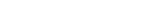where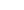angle of rotation
Angle of rotation
In mathematics, the angle of rotation is a measurement of the amount, the angle, that a figure is rotated about a fixed point, often the center of a circle....

,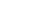rotation axis (unitary vector) and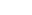pseudoscalar (trivector in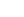## Properties

The Euler angles form a chart on all of SO(3), the special orthogonal group of rotations in 3D space. The chart is smooth except for a polar coordinate style singularity along β=0. See charts on SO(3)
Charts on SO(3)
In mathematics, the special orthogonal group in three dimensions, otherwise known as the rotation group SO, is a naturally occurring example of a manifold. The various charts on SO set up rival coordinate systems: in this case there cannot be said to be a preferred set of parameters describing a...

for a more complete treatment.

The space of rotations is called in general "The Hypersphere of rotations", though this is a misnomer: the group Spin(3) is isometric to the hypersphere S3, but the rotation space SO(3) is instead isometric to the real projective space
Real projective space
In mathematics, real projective space, or RPn, is the topological space of lines through 0 in Rn+1. It is a compact, smooth manifold of dimension n, and a special case of a Grassmannian.-Construction:...

RP3 which is a 2-fold quotient space
Quotient space
In topology and related areas of mathematics, a quotient space is, intuitively speaking, the result of identifying or "gluing together" certain points of a given space. The points to be identified are specified by an equivalence relation...

of the hypersphere. This 2-to-1 ambiguity is the mathematical origin of spin in physics
Spin (physics)
In quantum mechanics and particle physics, spin is a fundamental characteristic property of elementary particles, composite particles , and atomic nuclei.It is worth noting that the intrinsic property of subatomic particles called spin and discussed in this article, is related in some small ways,...

.

A similar three angle decomposition applies to SU(2), the special unitary group
Special unitary group
The special unitary group of degree n, denoted SU, is the group of n×n unitary matrices with determinant 1. The group operation is that of matrix multiplication...

of rotations in complex 2D space, with the difference that β ranges from 0 to 2π. These are also called Euler angles.

The Haar measure
Haar measure
In mathematical analysis, the Haar measure is a way to assign an "invariant volume" to subsets of locally compact topological groups and subsequently define an integral for functions on those groups....

for Euler angles has the simple form sin(β)dαdβdγ, usually normalized by a factor of 1/8π².

For example, to generate uniformly randomized orientations, let α and γ be uniform from 0 to 2π, let z be uniform from −1 to 1, and let β = arccos(z).

## Higher dimensions

It is possible to define parameters analogous to the Euler angles in dimensions higher than three.

The number of degrees of freedom of a rotation matrix is always less than the dimension of the matrix squared. That is, the elements of a rotation matrix are not all completely independent. For example, the rotation matrix in dimension 2 has only one degree of freedom, since all four of its elements depend on a single angle of rotation. A rotation matrix in dimension 3 (which has nine elements) has three degrees of freedom, corresponding to each independent rotation, for example by its three Euler angles or a magnitude one (unit) quaternion.

In SO(4)
SO(4)
In mathematics, the group of rotations about a fixed point in four-dimensional Euclidean space is denoted SO. The name comes from the fact that it is the special orthogonal group of order 4....

the rotation matrix is defined by two quaternions, and is therefore 6-parametric (three degrees of freedom for every quaternion). The 4x4 rotation matrices have therefore 6 out of 16 independent components.

Any set of 6 parameters that define the rotation matrix could be considered an extension of Euler angles to dimension 4.

In general, the number of euler angles in dimension D is quadratic in D; since any one rotation consists of choosing two dimensions to rotate between, the total number of rotations available in dimension D is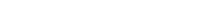, which for D=2,3,4 yields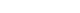.

## Applications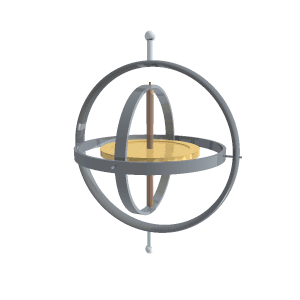### Vehicles and moving frames

Their main advantage over other orientation descriptions is that they are directly measurable from a gimbal mounted in a vehicle. As gyroscopes keep their rotation axis constant, angles measured in a gyro frame are equivalent to angles measured in the lab frame. Therefore gyros are used to know the actual orientation of moving spacecrafts, and Euler angles are directly measurable. Intrinsic rotation angle cannot be read from a single gimbal, so there has to be more than one gimbal in a spacecraft. Normally there are at least three for redundancy. There is also a relation to the well-known gimbal lock
Gimbal lock
Gimbal lock is the loss of one degree of freedom in a three-dimensional space that occurs when the axes of two of the three gimbals are driven into a parallel configuration, "locking" the system into rotation in a degenerate two-dimensional space....

problem of Mechanical Engineering
Mechanical engineering
Mechanical engineering is a discipline of engineering that applies the principles of physics and materials science for analysis, design, manufacturing, and maintenance of mechanical systems. It is the branch of engineering that involves the production and usage of heat and mechanical power for the...

.
The most popular application is to describe aircraft attitudes, normally using a Tait–Bryan convention so that zero degrees elevation represents the horizontal attitude. Tait–Bryan angles represent the orientation of the aircraft respect a reference axis system (world frame) with three angles which in the context of an aircraft are normally called Heading, Elevation and Bank. When dealing with vehicles, different axes conventions
Axes conventions
Mobile objects are normally tracked from an external frame considered fixed. Other frames can be defined on those mobile objects to deal with relative positions for other objects. Finally, attitudes or orientations can be described by a relationship between the external frame and the one defined...

are possible.

When studying rigid bodies in general, one calls the xyz system space coordinates, and the XYZ system body coordinates. The space coordinates are treated as unmoving, while the body coordinates are considered embedded in the moving body. Calculations involving acceleration
Acceleration
In physics, acceleration is the rate of change of velocity with time. In one dimension, acceleration is the rate at which something speeds up or slows down. However, since velocity is a vector, acceleration describes the rate of change of both the magnitude and the direction of velocity. ...

, angular acceleration
Angular acceleration
Angular acceleration is the rate of change of angular velocity over time. In SI units, it is measured in radians per second squared , and is usually denoted by the Greek letter alpha .- Mathematical definition :...

, angular velocity
Angular velocity
In physics, the angular velocity is a vector quantity which specifies the angular speed of an object and the axis about which the object is rotating. The SI unit of angular velocity is radians per second, although it may be measured in other units such as degrees per second, revolutions per...

, angular momentum
Angular momentum
In physics, angular momentum, moment of momentum, or rotational momentum is a conserved vector quantity that can be used to describe the overall state of a physical system...

, and kinetic energy
Kinetic energy
The kinetic energy of an object is the energy which it possesses due to its motion.It is defined as the work needed to accelerate a body of a given mass from rest to its stated velocity. Having gained this energy during its acceleration, the body maintains this kinetic energy unless its speed changes...

are often easiest in body coordinates, because then the moment of inertia tensor does not change in time. If one also diagonalizes the rigid body's moment of inertia tensor (with nine components, six of which are independent), then one has a set of coordinates (called the principal axes) in which the moment of inertia tensor has only three components.

The angular velocity of a rigid body takes a simple form using Euler angles in the moving frame. Also the Euler's rigid body equations are simpler because the inertia tensor is constant in that frame.### Others

Euler angles, normally in the Tait–Bryan convention, are also used in robotics
Industrial robot
An industrial robot is defined by ISO as an automatically controlled, reprogrammable, multipurpose manipulator programmable in three or more axes...

for speaking about the degrees of freedom of a wrist
Robotic arm
A mechanical arm is a robotic, usually programmable, with similar functions to a human arm. The links of such a manipulator are connected by joints allowing either rotational motion or translational displacement. The links of the manipulator can be considered to form a kinematic chain...

. They are also used in Electronic stability control
Electronic stability control
Electronic stability control is a computerized technology that may potentially improve the safety of a vehicle's stability by detecting and minimizing skids. When ESC detects loss of steering control, it automatically applies the brakes to help "steer" the vehicle where the driver intends to go...

in a similar way.

Gun fire control systems require corrections to gun-order angles (bearing and elevation) to compensate for deck tilt (pitch and roll). In traditional systems, a stabilizing gyroscope with a vertical spin axis corrects for deck tilt, and stabilizes the optical sights and radar antenna. However, gun barrels point in a direction different from the line of sight to the target, to anticipate target movement and fall of the projectile due to gravity, among other factors. Gun mounts roll and pitch with the deck plane, but also require stabilization. Gun orders include angles computed from the vertical gyro data, and those computations involve Euler angles.

Euler angles are also used extensively in the quantum mechanics of angular momentum. In quantum mechanics, explicit descriptions of the representations of SO(3) are very important for calculations, and almost all the work has been done using Euler angles. In the early history of quantum mechanics, when physicists and chemists had a sharply negative reaction towards abstract group theoretic methods (called the Gruppenpest), reliance on Euler angles was also essential for basic theoretical work.

In materials science, crystallographic texture
Texture (crystalline)
In materials science, texture is the distribution of crystallographic orientations of a polycrystalline sample. A sample in which these orientations are fully random is said to have no texture. If the crystallographic orientations are not random, but have some preferred orientation, then the...

(or preferred orientation) can be described using Euler angles. In texture analysis, the Euler angles provide the necessary mathematical depiction of the orientation of individual crystallites within a polycrystalline material, allowing for the quantitative description of the macroscopic material.
The most common definition of the angles is due to Bunge and corresponds to the ZXZ convention. It is important to note, however, that the application generally involves axis transformations of tensor quantities, i.e. passive rotations. Thus the matrix that corresponds to the Bunge Euler angles is the transpose of that shown in the table above.

Many mobile computing devices contain accelerometer
Accelerometer
An accelerometer is a device that measures proper acceleration, also called the four-acceleration. This is not necessarily the same as the coordinate acceleration , but is rather the type of acceleration associated with the phenomenon of weight experienced by a test mass that resides in the frame...

s which can determine these devices' Euler angles with respect to the earth's gravitational attraction. These are used in applications such as games, bubble level simulations, and kaleidoscope
Kaleidoscope
A kaleidoscope is a circle of mirrors containing loose, colored objects such as beads or pebbles and bits of glass. As the viewer looks into one end, light entering the other end creates a colorful pattern, due to the reflection off the mirrors...

s.

## See also

• Rotation representation
Rotation representation (mathematics)
In geometry a rotation representation expresses a rotation as a mathematical transformation. In physics, this concept extends to classical mechanics where rotational kinematics is the science of describing with numbers the purely rotational motion of an object.According to Euler's rotation theorem...

• Euler's rotation theorem
Euler's rotation theorem
In geometry, Euler's rotation theorem states that, in three-dimensional space, any displacement of a rigid body such that a point on the rigid body remains fixed, is equivalent to a single rotation about some axis that runs through the fixed point. It also means that the composition of two...

• Quaternions
• Axis angle
Axis angle
The axis-angle representation of a rotation, also known as the exponential coordinates of a rotation, parameterizes a rotation by two values: a unit vector indicating the direction of a directed axis , and an angle describing the magnitude of the rotation about the axis...

• Simultaneous orthogonal rotations angle
Simultaneous orthogonal rotations angle
A simultaneous orthogonal rotations angle is a vector representing angular orientation of a rigid-body relative to some reference frame...

• Conversion between quaternions and Euler angles
Conversion between quaternions and Euler angles
Spatial rotations in three dimensions can be parametrized using both Euler angles and unit quaternions. This article explains how to convert between the two representations. Actually this simple use of "quaternions" was first presented by Euler some seventy years earlier than Hamilton to solve the...

• Quaternions and spatial rotation
Quaternions and spatial rotation
Unit quaternions provide a convenient mathematical notation for representing orientations and rotations of objects in three dimensions. Compared to Euler angles they are simpler to compose and avoid the problem of gimbal lock. Compared to rotation matrices they are more numerically stable and may...

• Spherical coordinate system
Spherical coordinate system
In mathematics, a spherical coordinate system is a coordinate system for three-dimensional space where the position of a point is specified by three numbers: the radial distance of that point from a fixed origin, its inclination angle measured from a fixed zenith direction, and the azimuth angle of...

• Camera transform

## External links

• Java applet for the simulation of Euler angles available at http://www.parallemic.org/Java/EulerAngles.html.
• http://sourceforge.net/projects/orilib – A collection of routines for rotation / orientation manipulation, including special tools for crystal orientations. A generalization of Euler Angles to n-dimensional real spaces
The source of this article is wikipedia, the free encyclopedia.  The text of this article is licensed under the GFDL.